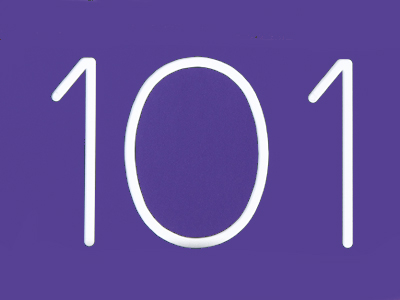The best way to multiply 93 by 101 is to multiply it by 100 and then add 93.

# Multiplication and Division (Year 6)

In KS2 Maths, you'll have loads of fun with multiplication and division! By Year Six, you'll be a times tables champ and a pro at basic multiplication and division. You'll also get to know cool words like 'multiple', 'factor', and 'product'.

Multiplication and division are super cool because they involve remainders – the bits left over. You'll learn to turn leftovers (remainders) into fractions and decimals. Imagine having 4 cats and 10 treats – you give each cat 2 treats, and you're left with 2 treats! Let's turn that into maths magic: 10 ÷ 4 = 2 r2 or 2.5 or 212. Now, test your awesome skills with a fun quiz about multiplication and division!

1.
Which strategy would be best to multiply 53 by 25?
Multiply by 100 then double
Multiply by 4 then 100
Multiply by 100 then divide by 4
Divide by 4 then double
Just as 0.25 is 14 of 1, 25 is 14 of 100
2.
5.87 x 100 = ?
587
5,870
0.587
0.0587
To multiply a number by 100 move the digits 2 places to the left
3.
How could we find an eighth of a number?
Multiply it by 4
Multiply it by 8
Divide it by 8
Divide it by 4
An eighth of a number is the same as saying divide it by 8
4.
2.43 x 10 = ?
2.430
24.3
243
0.243
To multiply a number by 10 move the digits 1 place to the left
5.
(6 + 5) x (8 - 3) + 6 =
(6 + 5 x 8) - (3 + 6) =
6 + 5 x (8 - 3) + 6 =
(6 + 5) x 8 - 3 + 6 =
Work out the calculations in brackets first
6.
On a calculator the answer to a division question is 5.25. What fraction is the remainder?
Half
Quarter
Third
Eighth
0.25 is the decimal equivalent of 14
7.
Which of these methods would result in finding 112?
Half one third twice
Half one quarter twice
Double one quarter twice
Double one sixth
e.g. 112 of 66. One third is 22, half of 22 is 11, half of 11 is 5.5
8.
Which strategy would be best to multiply 93 by 101?
Multiply by 10 then add 93
Multiply by 100 then add 93
Multiply by 100 then subtract 93
Multiply by 10 then subtract 93
You can use this strategy for other numbers over 100, for example to multiply a number by 107 multiply by 100 and also by 7 then add the 2 results together
9.
What is the best method of multiplying 81 by 99?
Multiply by 100 then subtract 81
Multiply by 10 then add 81
Multiply by 100 then add 81
Multiply by 10 then subtract 81
Multiplying by 100 is much easier than multiplying by 99
10.
What is the best method of multiplying 72 by 15?
Multiply by 10 then halve
Multiply by 10, halve the result, then add the two parts together
Multiply by 10, divide by 5, then add the two numbers
Divide by 10 then double
72 x 10 = 720; half of 720 = 360, so 720 + 360 = 1,080